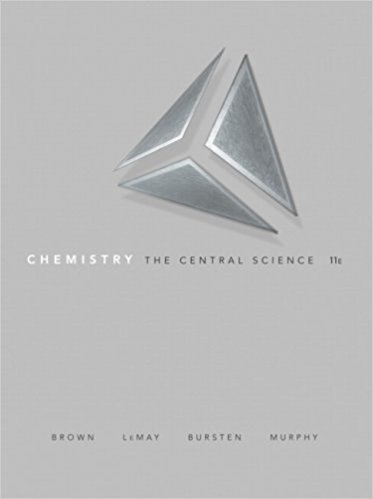×
×

# Consider the gas-phase transfer of an electron from a sodium atom to a chlorine atomISBN: 9780136006176 365

## Solution for problem 7.108 Chapter 7

Chemistry: The Central Science | 11th Edition

• Textbook Solutions
• 2901 Step-by-step solutions solved by professors and subject experts
• Get 24/7 help from StudySoup virtual teaching assistantsChemistry: The Central Science | 11th Edition

4 5 1 408 Reviews
13
2
Problem 7.108

Consider the gas-phase transfer of an electron from a sodium atom to a chlorine atom: Na{g) + Cl{g) ----> Na+{g) + Cl-{g) (a) Write this reaction as the sum of two reactions, one that relates to an ionization energy and one that relates to an electron affinity. (b) Use the result from part (a), data in this chapter, and Hess's law to calculate the enthalpy of the above reaction. Is the reaction exothermic or endothermic? (c) The reaction between sodium metal and chlorine gas is highly exothermic and produces NaCl(s), whose structure was discussed in Section 2.7. Comment on this observation relative to the calculated enthalpy for the aforementioned gas-phase reaction.

Step-by-Step Solution:
Step 1 of 3

Spencer Kociba CHEM 101 Lecture 11/15/16 THERMOCHEMISTRY ● Enthalpy ○ Represented as ΔH ○ Internal energy + pressure*volume ■ We will mostly be concerned with constant pressure. This is where the formula ΔH = ΔE + PΔV comes from ○ ΔH = q (enthalpy=heat gained or lost in the system) ● Properties of Enthalpy (ΔH ) ○...

Step 2 of 3

Step 3 of 3

##### ISBN: 9780136006176

Unlock Textbook Solution